•• Merch
• Donate
• SAT Prep

IntroductionIntroduction# Volume of Cylinders and Prisms

INTRO
Volume tells us the amount of space there is inside a 3D shape. It’s kind of like the 3D version of area 🤯. To find the volume of a cylinder or prism, we multiply the area of the base by the height.To help us understand why this is the formula for volume, let’s imagine we have a cake with lots of layers.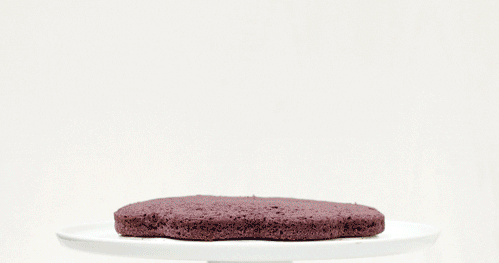CALCULATOR

## Volume of Cylinders & Prisms Calculator

#### Step 1. Imagine & identify the base.

We can think of the cylinder as a cake with layers shaped as circles. The base is the bottom layer, a circle.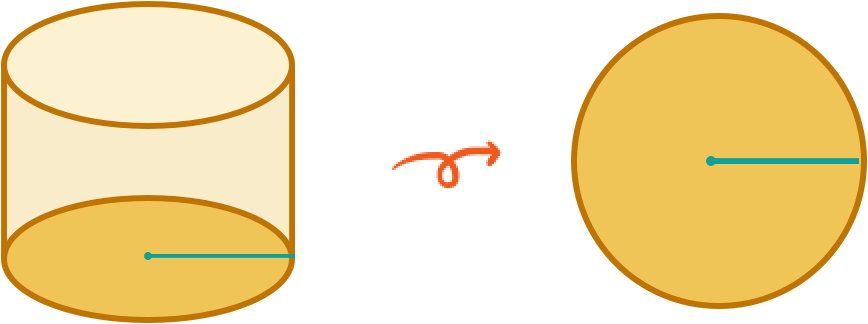KEY STEPS

## How to Find the Volume of Cylinders & Prisms

Shape:

### Step 1. Imagine & identify the base.

We can think of the cylinder as a cake with layers shaped as circles. The base is the bottom layer, a circle.

### Step 2. Calculate the area of the base.

We start by calculating the area of the base circle.

### Step 3. Multiply the area of the base by the height (H) of the cylinder.

Then, we multiply the base area by the height to get the volume.
LESSON
Volume of Cylinders & Prisms

## Volume of Cylinders & Prisms

The two main things we need to know in order to solve for the volume of a cylinder and prism are the area of the base and the height of the 3D shape.Once we have those two values all that’s left is to multiply the base area by the height, and then we're set! 👌🏿👌🏽👌🏻
In cake terms, the base area is like the amount of cake in one layer, and the height is like the total number of layers in the cake.
When we multiply the base area by the height, we can think of this step as stacking the layers of the cake until we reach the desired height.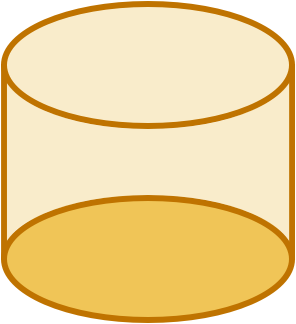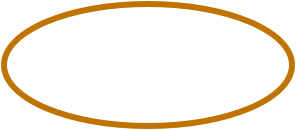PRACTICE
Volume of Cylinders & Prisms

## Practice: Volume of Cylinders & Prisms

Question 1 of 3: Find the volume of this cylinder ⬇️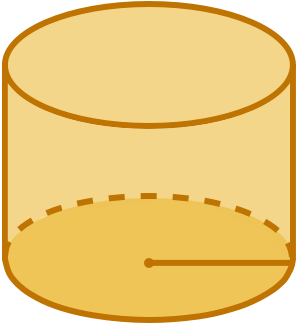### Step 1. Imagine & identify the base.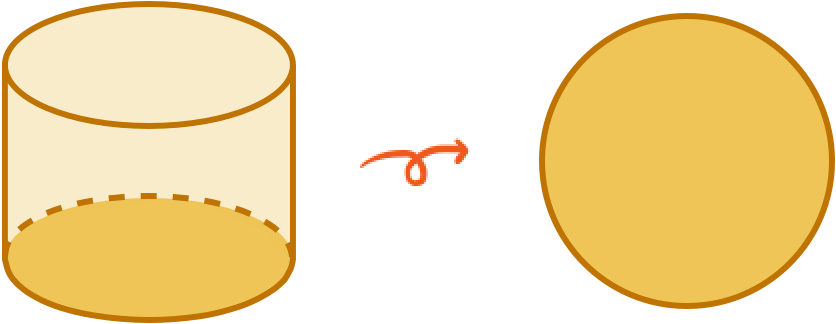The base of a cylinder is a.

CONCLUSION
Nice work, look at you go! Thanks for checking out this lesson ☺️🙏. Where to next?Leave Feedback